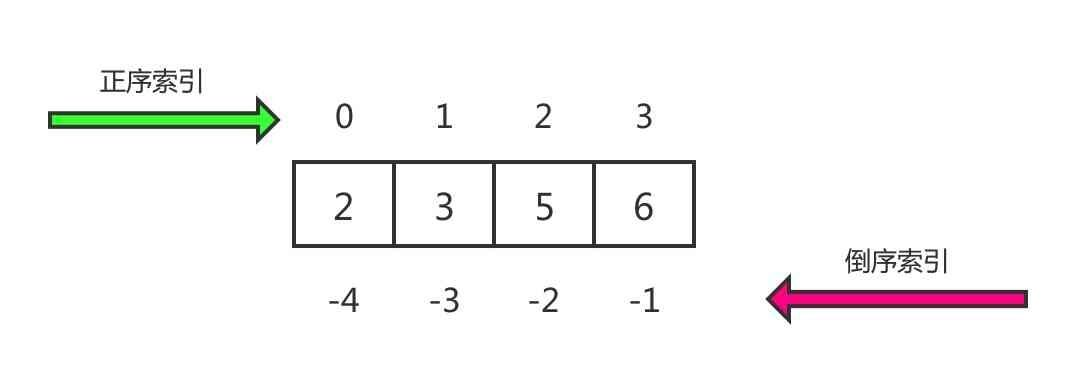# python基础数据类型列表(list)详解

## 1. python列表定义

python的列表是一种有序且可变的序列，列表使用中括号[]进行定义，各项元素之间使用逗号分隔。python的列表与其他编程语言中的数组很像，但独特之处在于python并不要求列表中的元素必须都是同一个类型，而是可以存储任意类型的数据。

## 1. 创建列表

1. 使用[] 创建列表
2. 使用内置函数创建列表

``````empty_list = []         # 创建一个空列表
``````

``````lst = [1, '2', True, [1, 2]]
``````

``````lst1 = list("python")
lst2 = list([1, 2, 3])
print(lst1)     # ['p', 'y', 't', 'h', 'o', 'n']
print(lst2)     # [1, 2, 3]
``````

## 2. 访问列表里的元素

``````list[index]
``````

``````lst = [2, 3, 5, 6]
````````````print(lst)       # 6
print(lst[-1])      # 6
``````

## 3. 向列表里新增数据

1. append
2. insert
3. extend

append方法在列表的末尾新增数据

``````lst = [1, 2, 3]
lst.append(4)
print(lst)          # [1, 2, 3, 4]
``````

insert方法在指定的索引位置的前面向列表中插入一个新的元素，指定的插入索引位置是多少，新元素的索引就是多少。

``````lst = [1, 2, 3]
lst.insert(0, 4)
print(lst)      # [4, 1, 2, 3]
``````

append和inset一次只能新增一个元素，extend方法可以一次性将另一个序列里的元素追加到列表的末尾

``````lst1 = [1, 2, 3]
lst2 = [4, 5, 6]

lst1.extend(lst2)
print(lst1)         # [1, 2, 3, 4, 5, 6]
``````

## 4. 修改列表里的数据

``````lst = [1, 2, 3]
lst = 100
print(lst)      # [100, 2, 3]
``````

## 5. 删除列表中的数据

1. remove
2. pop
3. del
4. clear

``````lst = [1, 2, 3, 2]
lst.remove(2)       # 明确指明要删除2
print(lst)          # [1, 3, 2]
``````

``````lst = [1, 2, 3, 2]
lst.pop(2)       # 指明要删除索引为2的元素
print(lst)          # [1, 2, 2]
``````

``````lst = [1, 2, 3, 2]
del lst
print(lst)    # [1, 2, 2]
``````

clear方法清空列表，删除所有元素

``````lst = [1, 2, 3, 2]
lst.clear()
print(lst)      # []
``````

clear方法情况列表后，lst变为一个空列表。

## 6. 遍历列表

``````lst = [1, 2, 3, 2]

for item in lst:
print(item)
``````

``````1
2
3
2
``````

## 7 列表的一些基础常规操作

``````lst = [1, 2, 3, 2]

print(len(lst))     # 4
``````

``````lst = [1, 2, 3, 2]

for i in range(len(lst)):
print(lst[i])
``````

python的序列，例如字符串，列表，元组，都支持算数运算符+

``````lst1 = [1, 2, 3]
lst2 = [4, 5, 6]

lst3 = lst1 + lst2
print(lst3)     # [1, 2, 3, 4, 5, 6]
``````

``````lst1 = [1, 2, 3]
lst2 = lst1*3
print(lst2)      # [1, 2, 3, 1, 2, 3, 1, 2, 3]
``````

lst1*3，会生成一个新的列表，新列表的长度是lst1的3倍。

``````lst1 = [1, 2, 3]
print(2 in lst1)            # True
print(3 not in lst1)        # False
``````

in 可用于判断一个元素是否在列表中，如果前面加一个not，就表示判断一个元素是否不在列表中。

## 8. 列表的常用方法

count() 统计某个元素在列表中出现的次数
append() 在列表末尾添加新的对象
extend 在列表末尾一次性追加另一个序列中的多个值
index 返回一个元素在列表中第一次出现的位置索引
insert() 将对象插入列表中的指定位置
pop() 删除列表中指定索引的元素，默认删除最后一个并返回该值
remove() 移除列表中某个值的第一个匹配项
reverse() 翻转列表
sort() 对列表进行排序QQ交流群: 211426309